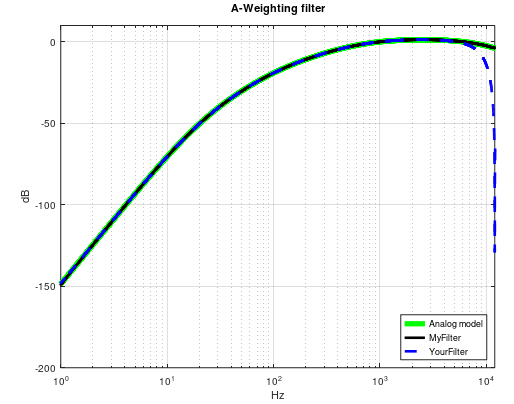Started by 2 years ago3 replieslatest reply 2 years ago246 views

I have tested a few filters, and they all appear to work with the above functions that I want to use, but the following filter does not work as inteded. The q32 coefficients below does work with the q32 functions, and the coefficients are converted from the float coefficients to q32 and q15 coefficients using the same scritpt every time.

Question:
With the q15 coefficients below, the filtered signal becomes more and more negative with every sample like this. Does that mean anything to anyone? The coefficients should not be to large? Rounding error?A-weighting float filter coefficients (b0, b1, b2, -a1, -a2):

[ 0.42562639  0.85125278  0.42562639  0.45929809  0.05273868

1.         -2.0001525   1.00015251 -1.7960507   0.80094643

1.         -1.9998475   0.99984751 -1.98924339  0.98927232]

q32 coefficients (b0, b1, b2, a1, a2) (post shift = 2 -> all coefficients are divided by 4):

[  228506428   457012856   228506428  -246583783   -28313865

536870912 -1073823698   536952792   964247376  -430004838

536870912 -1073659950   536789045  1067966915  -531111533]

q15 coefficients (b0, 0, b1, b2, a1, a2) (post shift = 2 -> all coefficients are divided by 4):

[  3487      0   6973   3487  -3763   -432

8192      0 -16385   8193  14713  -6561

8192      0 -16383   8191  16296  -8104]

From the doc:
The zero coefficient between b1 and b2 facilities use of 16-bit SIMD instructions on the Cortex-M4

[ - ]You're always looking at the ratio of the largest coefficient to the smallest.  Too big, and you start to get into problems, because after dividing down to get all coefficients into the word size, the smaller coefficients don't have enough bits to be accurately represented - which I've seen as DC offset on a filter response, but could manifest itself in other ways, possibly the increasing negative response you're seeing.

Between your largest and smallest, the ratio is about 38.  That's quite a stretch to do in a 16 bit word possibly, whereas the additional 16 bits of the "32 bit" versions of the filter has more bits to accurately represent that wide range of the coefficients.

You can code up the same in a C routine (always recommended as first step).  In one case, use int32_t for the coefficients, with the 32 bit versions, in the other use int16_t, for the 15 bit ones.  Run your data set through, look at the output.

Regards,

Robert

[ - ]I haven't checked the exact implementation, but I had trouble with this before:

You have one coefficient smaller than -2 (-2.0001525) - it might be that this causes an overflow somewhere down the line. To check if this is the problem, just change the coefficient to -1.999 and run those coefs both in CMSIS and MATLAB or whatever you use. If the results compare well you have the trouble maker (NOTE: this only tells you if the overflow was the problem - changing the coeff might render the filter useless, of course)

[ - ]Your A-Weighting filter is maybe std BLT implementation and therefore has some error in response at high frequency area :Dunno if your issue relates to this difference so, I prepared these (fs=24kHz), closer to analog response, coefficients so you can try if it helps in your issue (sorry but I did not have time to convert these to Q format):

sos =

Columns 1 through 5:

1.000000000000000e+00  -1.999999983069380e+00   9.999999975125764e-01   1.000000000000000e+00  -1.989243394531790e+00
1.000000000000000e+00   8.442076357132433e-01   1.252234426103703e-01   1.000000000000000e+00   5.525233039464380e-01
1.000000000000000e+00  -2.000000016930624e+00   1.000000002487429e+00   1.000000000000000e+00  -1.796050696683829e+00

Column 6:

9.892723206721504e-01
-2.924321030469864e-02
8.009464255452426e-01

g = 0.868993330876026

! 3rd b1 < -2 so, depending on Q-format, overflow may happen
https://chummersone.github.io/qformat.html

If you need A-weighting filter for other sample rates then here's the method I used to calculate.